Next: ReferencesGeneral Bibliography and Up: Formulas of General Interest Previous: Formula for the Surface

# Formula to compute compound interest.

Here's a formula which can be used in 123, Excel, Wings and Dynaplan:

```     ------- Input this data -------------------------------
principal amount = E9                  ( in dollars )
Amortization Period = d10              ( in years ie 6 mon = .5 )
Payments / year = D11                  ( 12 = monthly, 52 = weekly )
Published Interest rate = D12          ( ie 9 % = 0.09 )
Times per year Int calculated = d13    ( CDN mortgage use 2
US mortgage use 12
all other loans use 12 )
----- Calculate the proper rate of interest -----------

e14 = Effective annual rate = EXP(D13*LN(1+(D12/D13)))-1
e15 = Interest rate per payment = (EXP(LN(E14+1)/(D10*D11))-1)*D10*D11

e17 = Payments = APMT(E9,E15/D11,D10*D11) ( both these functions are
= PMT (E9,E15/D11,D10*D11) ( identical,diff spreadsheet)
APMT( principal amount,interest rate per period,# periods )
( this is a standard function on any true commercial spreadsheet)

OR use the following if done using a calculator
= Payments = P*I/[1-(I+1)^-T]
= E9*(E15/D11)/(1-((E15/D11) +1)**(-1*D10*D11))

Total interest cost = E17*D10*D11-E9

-- Use these formulas if you wish to generate an amortization table --
always add up to 'Payments (e17)'
Interest per payment  = current balance * ( E15 / D11 )
Principal per payment = current balance - Interest per payment
new current balance   = current balance - Principal per payment -
(extra payment)

keep repeating until 'new current balance' = 0```

Derivation of Compound Interest Rate Formula

Suppose you deposited a fixed payment into an interest bearing account at regular intervals, say monthly, at the end of each month. How much money would there be in the account at the end of the nth month (at which point you've made n payments)?

Let i be the monthly interest rate as a fraction of principle.
Let x be the amount deposited each month.
Let n be the total number of months.
Let p[k] be the principle after k months.

So the recursive formula is: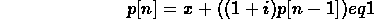This yields the summation: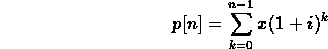The way to solve this is to multiply through by (1 + i) and subtract the original equation from the resulting equation. Observe that all terms in the summation cancel except the last term of the multiplied equation and the first term of the original equation: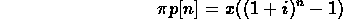or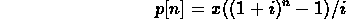Now suppose you borrow p at constant interest rate i. You make monthly payments of x. It turns out that this problem is identical to taking out a balloon loan of p (that is it's all due at the end of some term) and putting payments of x into a savings account. At the end of the term you use the principle in the savings account to pay off the balance of the loan. The loan and the savings account, of course, must be at the same interest rate. So what we want to know is: what monthly payment is needed so that the balance of the savings account will be identical to the balance of the balloon loan after n payments?

The formula for the principal of the balloon loan at the end of the nth month is: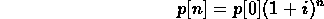So we set this expression equal to the expression for the the savings account, and we get: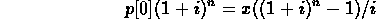or solving for x: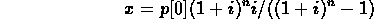If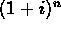is large enough (say greater than 5), here is an approximation for determining n from x, p, and i: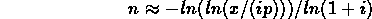The above approximation is based upon the following approximation: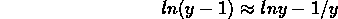Which is within 2 For example, a \$100000 loan at 1% monthly, paying \$1028.61 per month should be paid in 360 months. The approximation yields 358.9 payments.

If this were your 30 year mortgage and you were paying \$1028.61 per month and you wanted to see the effect of paying \$1050 per month, the approximation tells you that it would be paid off in 303.5 months (25 years and 3.5 months). If you stick 304 months into the equation for x, you get \$1051.04, so it is fairly close. This approximation does not work, though, for very small interest rates or for a small number of payments. The rule is to get a rough idea first of whatis. If that is greater than 5, the approximation works pretty well. In the examples given,is about 36.

Finding i given n, x, and p is not as easy. If i is less than 5% per payment period, the following equation approximately holds for i: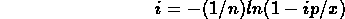There is no direct solution to this, but you can do it by Newton-Raphson approximation. Begin with a guess, i. Then apply: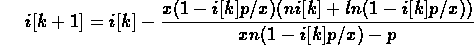You must start with i too big, because the equation for i has a solution at i=0, and that's not the one you want to end up with.

Example: Let the loan be for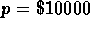,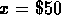per week for 5 years (n=260). Let i = 20% per annum or 0.3846% per week. Since i must be a fraction rather than a percent, i = 0.003846. Then, applying eq 11: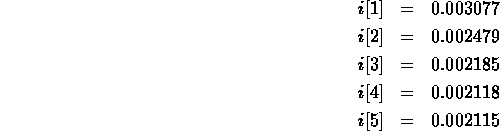The series is clearly beginning to converge here.

To get i as an annual percentage rate, multiply by 52 weeks in a year and then by 100%, so i = 10.997% per annum. Substituting i back into eq 7, we get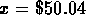, so it works pretty well.

References

The theory of interest. Stephen G. Kellison. Homewood, Ill., R. D. Irwin, 1970.Next: ReferencesGeneral Bibliography and Up: Formulas of General Interest Previous: Formula for the Surface

Alex Lopez-Ortiz
Mon Feb 23 16:26:48 EST 1998